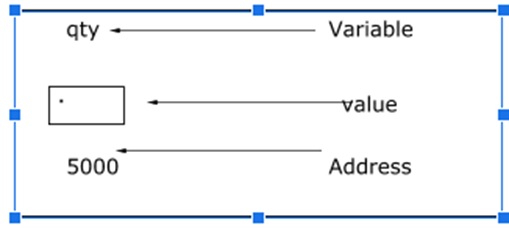Explain array of pointers in C programming language

CServer Side ProgrammingProgramming

Pointer is a variable that stores the address of another variable.

Features

• Pointer saves the memory space.
• Execution time of pointer is faster because of direct access to memory location.
• With the help of pointers, the memory is accessed efficiently, i.e., memory is allocated and deallocated dynamically.
• Pointers are used with data structures.

Pointer declaration and initialization

Consider the following statement −

int qty = 179;

In memory, the variable can be represented as follows −Declaring a pointer

It means ‘p’ is a pointer variable, which holds the address of another integer variable, as shown below −

Int *p;

Initialization of a pointer

Address operator (&) is used to initialise a pointer variable.

For Example − int qty = 175;

int *p;

p= &qty;

Array of pointers

It is collection of addresses (or) collection of pointers

Declaration

Following is the declaration for array of pointers −

datatype *pointername [size];

For example,

int *p;

It represents an array of pointers that can hold 5 integer element addresses.

Initialization

‘&’ is used for initialisation.

For Example,

int a = {10,20,30};
int *p, i;
for (i=0; i<3; i++) (or) for (i=0; i<3,i++)
p[i] = &a[i];
p[i] = a+i;

Accessing

Indirection operator (*) is used for accessing.

For Example,

for (i=0, i<3; i++)
printf ("%d", *p[i]);

Example program

Given below is the program for array of pointers −

Live Demo

#include<stdio.h>
main ( ){
int a = {10,20,30};
int *p,i;
for (i=0; i<3; i++)
p[i] = &a[i];
printf ("elements of the array are \n");
for (i=0; i<3; i++)
printf ("%d \t", *p[i]);
}

Output

When the above program is executed, it produces the following result −

elements at the array are : 10 20 30

Example 2

Given below is the program for the array of pointers to strings −

Live Demo

#include <stdio.h>
#include <stdlib.h>
int main(void){
char *a = {"one", "two", "three", "four", "five"};
int i;
printf ( "the strings are at locations:\n");
for (i=0; i<5; i++)
printf ("%d\n", a[i]);
return 0;
}

Output

When the above program is executed, it produces the following result −

The strings are at locations:
4210688
4210692
4210696
4210702
4210707